Practical Statistics for Data Scientists
Share
Explore
5. Classification

#Evaluating classification models

Accuracy
The percent/proportion of cases classified correctly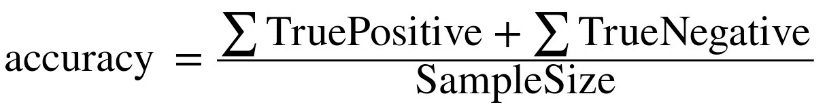Confusion matrix
A tabular display of the record counts by their predicted and actual classification status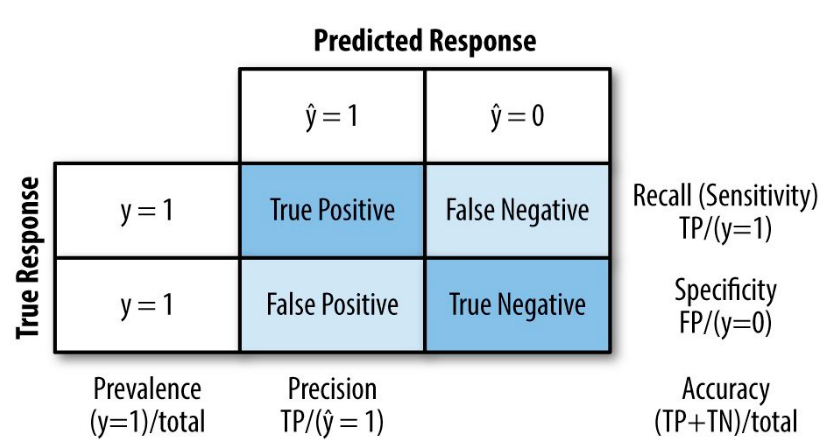The rare class problem
Depending on the relative cost, need to make the trade-off between false positives and false negatives
Precision, recall, and specificity
Search
Term
Description
Interpretation
Formula
R code
1
Specificity
The percent/proportion of 0s correctly classified
Measures a model's ability to predict a negative outcome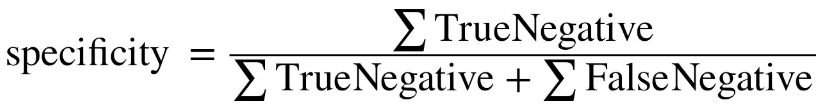conf_mat[2,2]/sum(conf_mat[2,])
2
Precision
The percent/proportion of predicted 1s that are actually 1s
The accuracy of a predicted positive outcome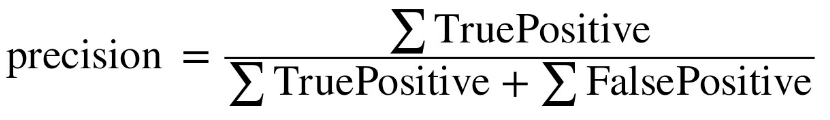conf_mat[1,1]/sum(conf_mat[,1])
3
Sensitivity/Recall
The percent/proportion of 1s correctly classified
Measure the strength of the model to predict a positive outcome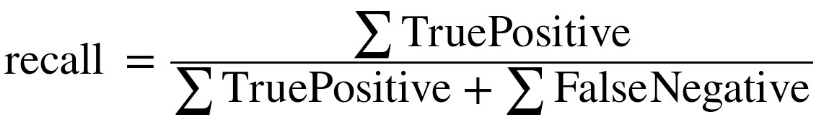conf_mat[1,1]/sum(conf_mat[1,])
There are no rows in this table
3
Count

A plot of trade-off between sensitivity and specificity as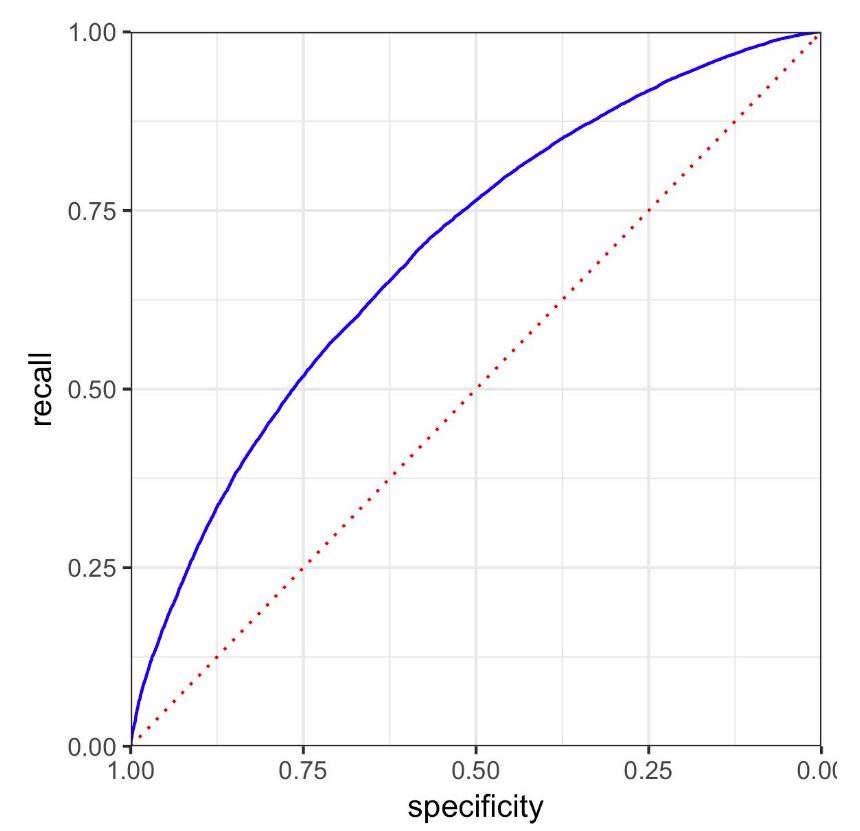Precision-recall curve

Area under the curve (AUC)

Lift
A measure of how effective the model is at identifying (comparatively rare) 1s at different probability cutoffs

Share